# Extremal set

(diff) ← Older revision | Latest revision (diff) | Newer revision → (diff)

A collection of solutions of the Euler equation, depending onarbitrary constants and filling without mutual intersections some part of the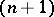-dimensional space. Hereis the number of unknown functions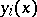,, on which the functional to be minimized,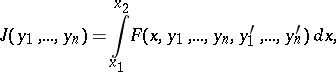depends. Euler's equation is understood in the vector sense, that is, it is a system ofordinary differential equations of the second order: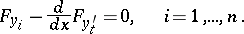Two methods for constructing an extremal set are indicated below.

Let the object of investigation be a pencil of extremals emanating from a given pointin an-dimensional space. If the extremals of the pencil do not intersect each other in some neighbourhood of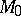(except at), then they form an extremal set (a central extremal set) in this neighbourhood.

Another method of constructing extremals consists in constructing the set of extremals that are transversal to a surface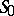given in the-dimensional space by an equation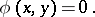If at every point of this surface the transversality conditions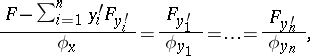in total, determine the value of thederivatives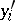,, then by taking these values as initial values of the derivatives one can draw through a point ofan extremal that intersectstransversally. If in a neighbourhood of this surface the above extremals do not intersect each other, then they form an extremal set (an ordinary, or proper extremal set).

The construction of an extremal set is the starting point in the discussion of questions connected with the construction of a field of extremals (cf. Extremal). An extremal set is an extremal field if there is a one-parameter family of surfaces that intersect the extremals of the set transversally.

How to Cite This Entry:
Extremal set. Encyclopedia of Mathematics. URL: http://encyclopediaofmath.org/index.php?title=Extremal_set&oldid=43478
This article was adapted from an original article by I.B. Vapnyarskii (originator), which appeared in Encyclopedia of Mathematics - ISBN 1402006098. See original article